Follow

Publishing view

There are three different categories when you want to publish a test result, Exam, Questions and Students Answers. Under these categories, you can choose options that should be visible or not to the students when they should see their published results.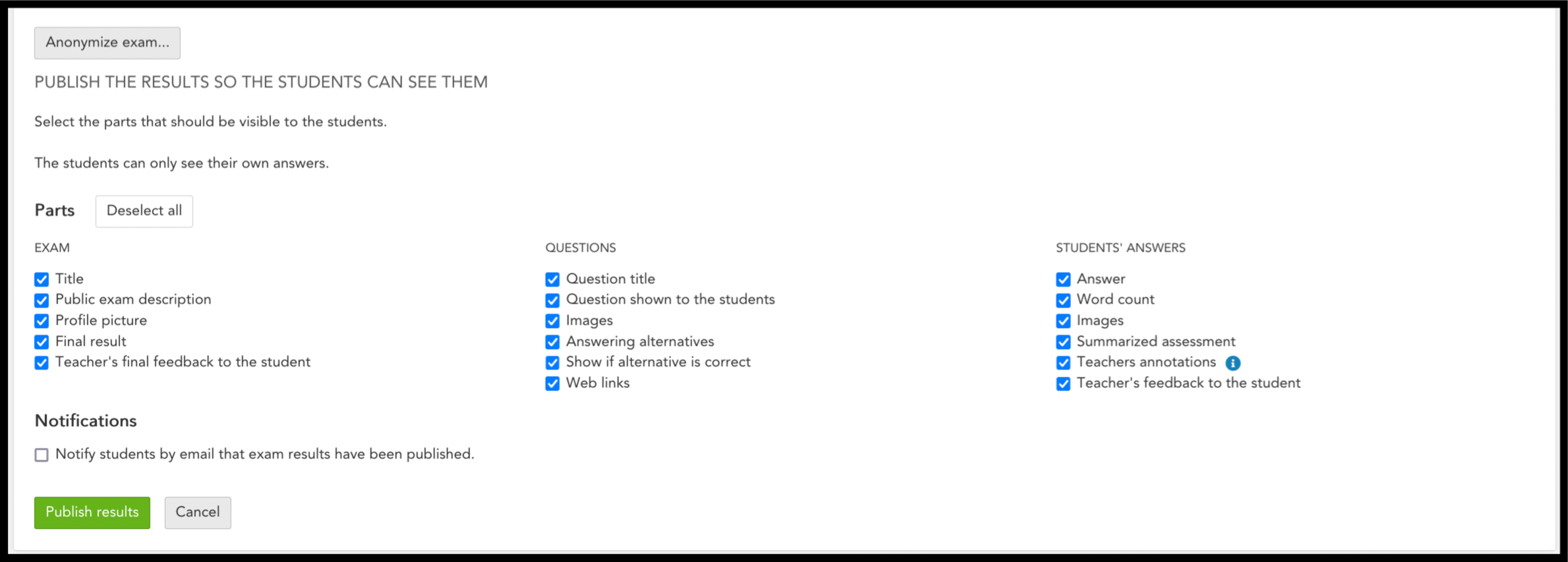Exam

Here, you can easily choose whether the students should be able to see the information they have given about the exam. For example, they can see the public exam description that you can add when creating the exam template.

Title

Title of the Exam

Public exam description

The exam description that you wrote whilst making the template.

Final result

The result that the student got on this exam.

Teacher's final feedback to the student

The comment that you as a teacher give the student when you grade the test. This is filled in a comment field below the score that you can edit on each question.

Examples of what these settings might look like: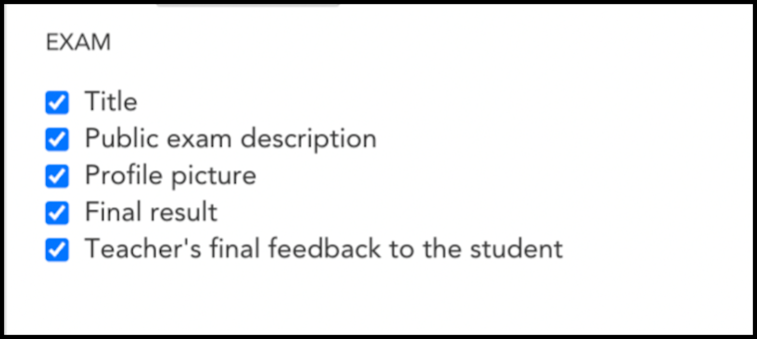Figure 1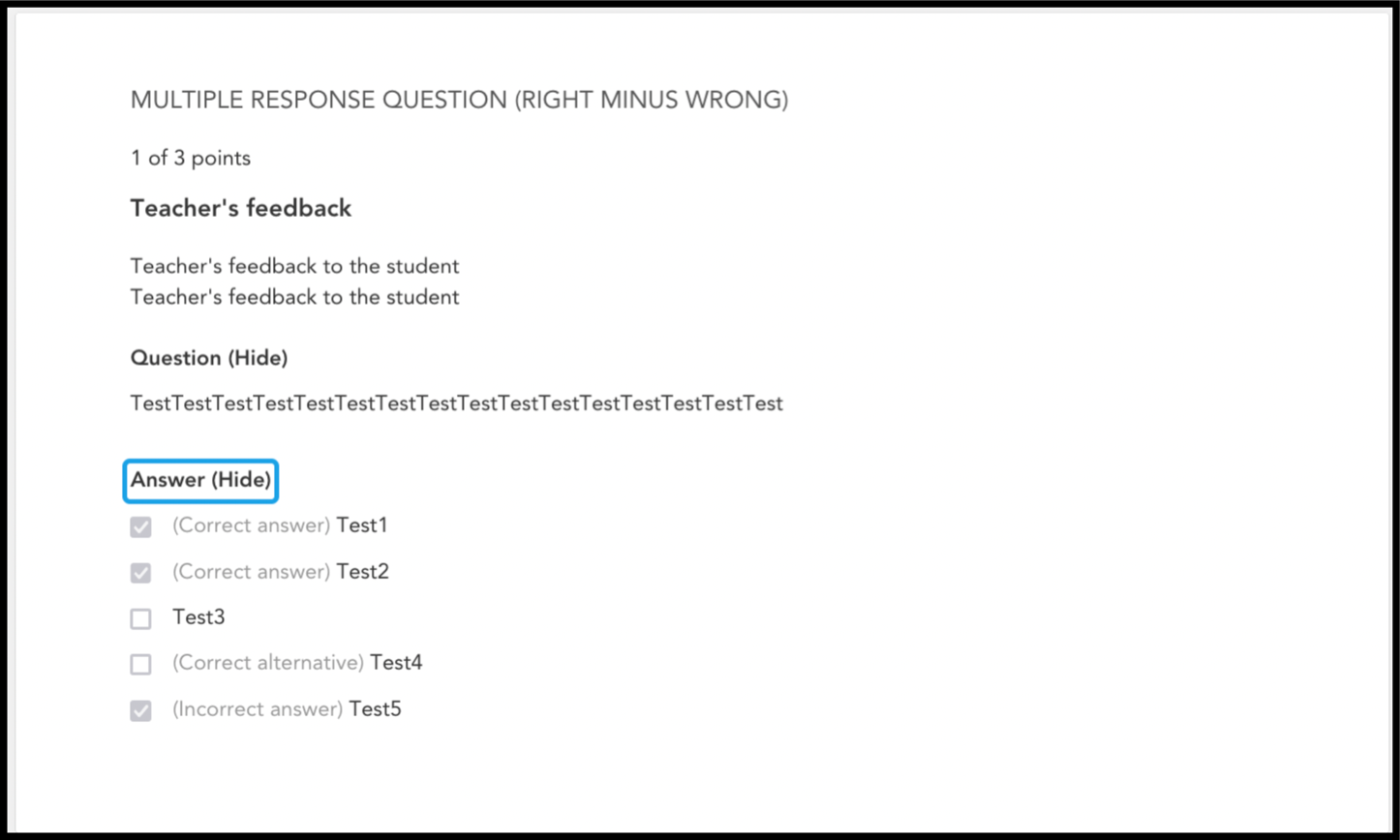Figure 2Figure 3

Questions

Here you have alternatives that are related to the exam itself, to the exam template that you have created. You can easily choose how much of the actual exam that the students have written should be visible to them. This is especially useful if you are going to reuse the exam template with the questions on several occasions, then you can uncheck options like the question itself, correct answer, etc.

Question title

The title / name of each question. For example, "Question 1".

Question shown to the students

The wording, the question that is asked and must be answered by the student.

Images

Any images that the students had in the test as an aid.

This applies to Multiple choice questions or Multiple correct answer questions. If you choose to publish this, the student sees all the alternatives they have had on the test.

Show if alternative is correct

This is linked to "Answer options" as you can choose whether you want the students to see what the correct answer is or not. If you choose to check this, the student only sees the score.

Examples of what these settings might look like: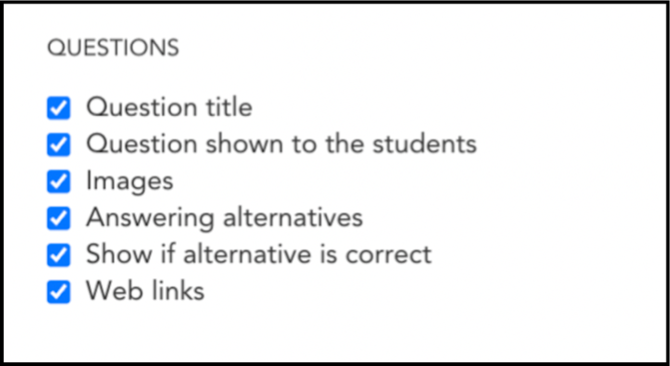Figure 4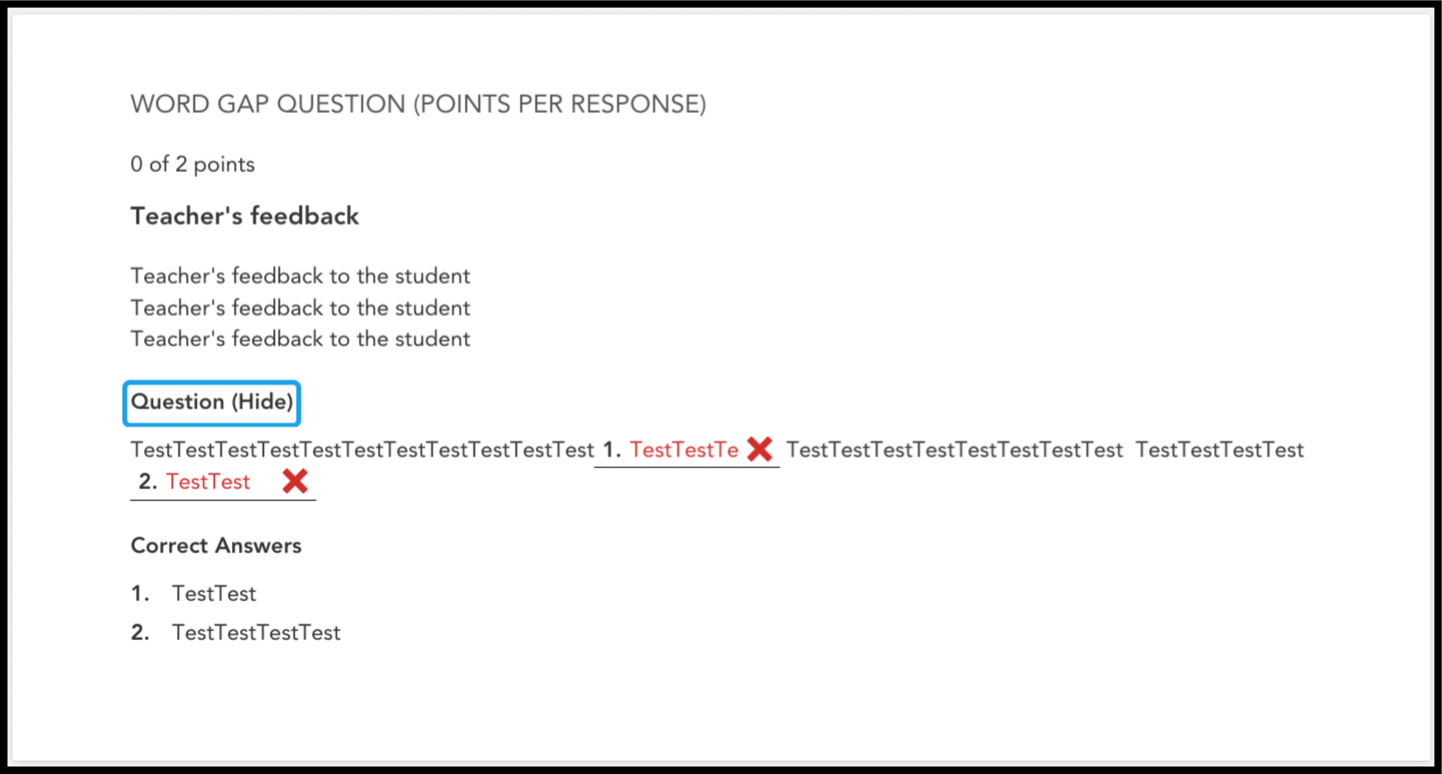Figure 5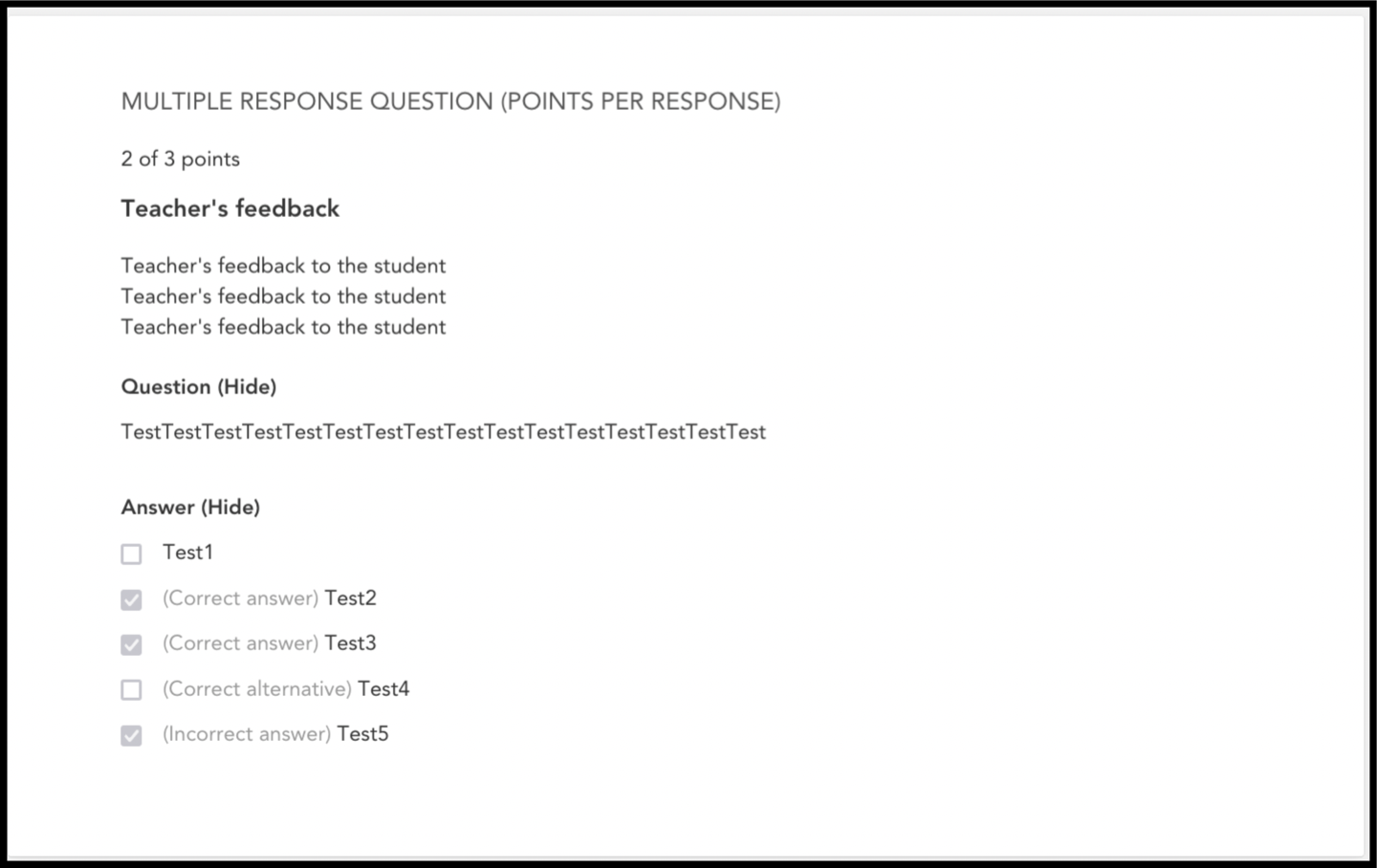Figure 6

This shows students what they have answered exactly, whether it is a text question, choice or word gap.

Word count

This shows students how many words they have written.

Images

Pictures that students have drawn / created in the test with our drawing tool.

Summarized assessments

Total score or grade on the test.

Teachers annotations

This shows the teacher's comment that can be created on certain words / sentences with colour markings.

Teacher's feedback to the student

Finally, this is the teacher's comment / feedback to the student.

Examples of what these settings might look like: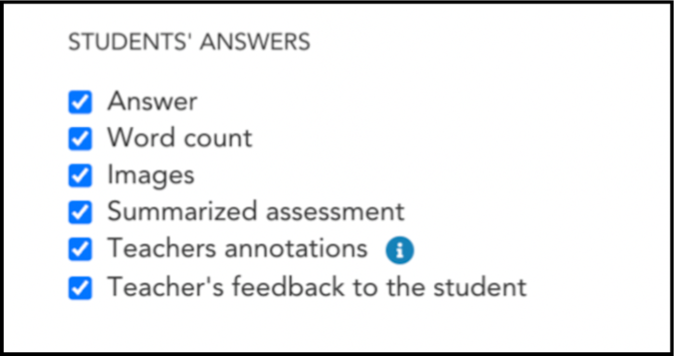Figure 7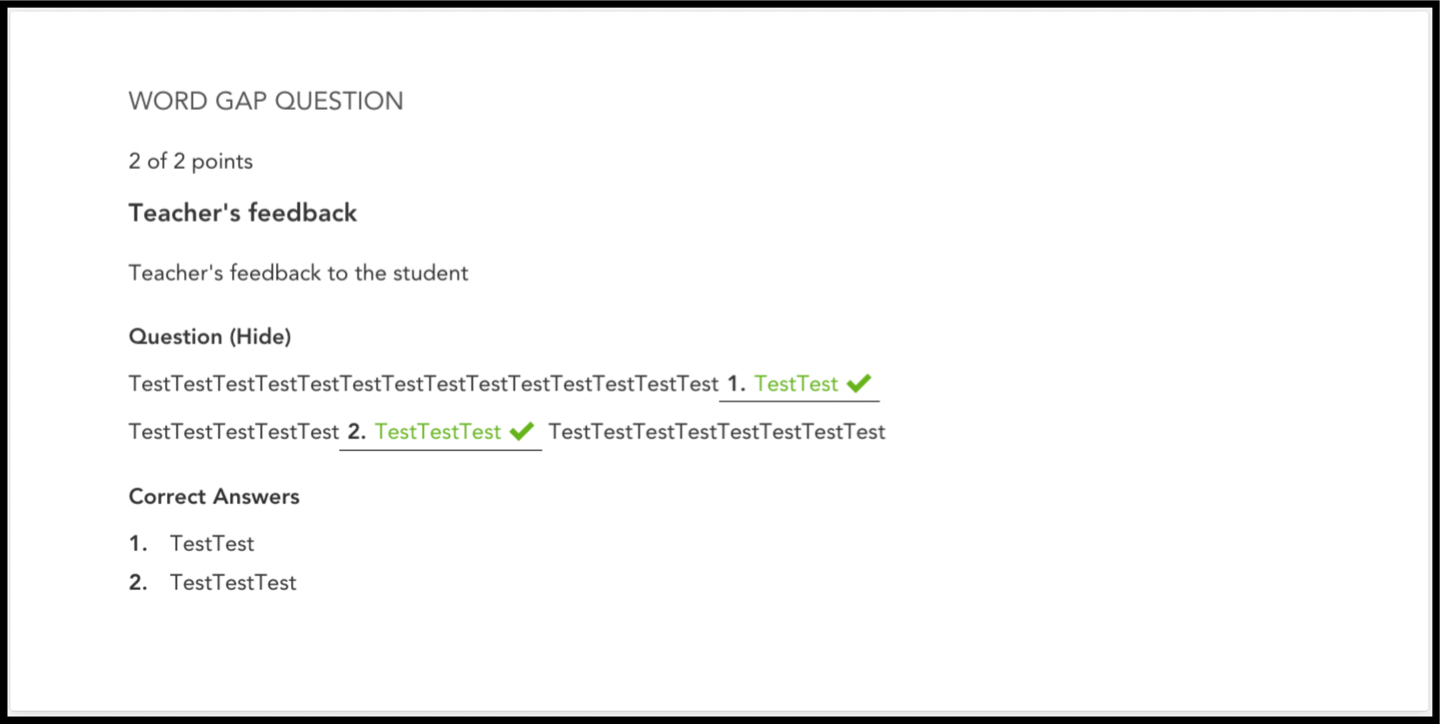Figure 8

Different examples of what it can look like with different settings:

Example one:

In figure nine, we can also see that "Notify students by email that exam results have been published." is chosen. This means that students will be notified via email that the results have been published. If you do not check it, the students will not know that the results have been published until they log in to DigiExam on the web or until you as a teacher inform the students of this.Figure 9Figure 10Figure 11

Example two:Figure 12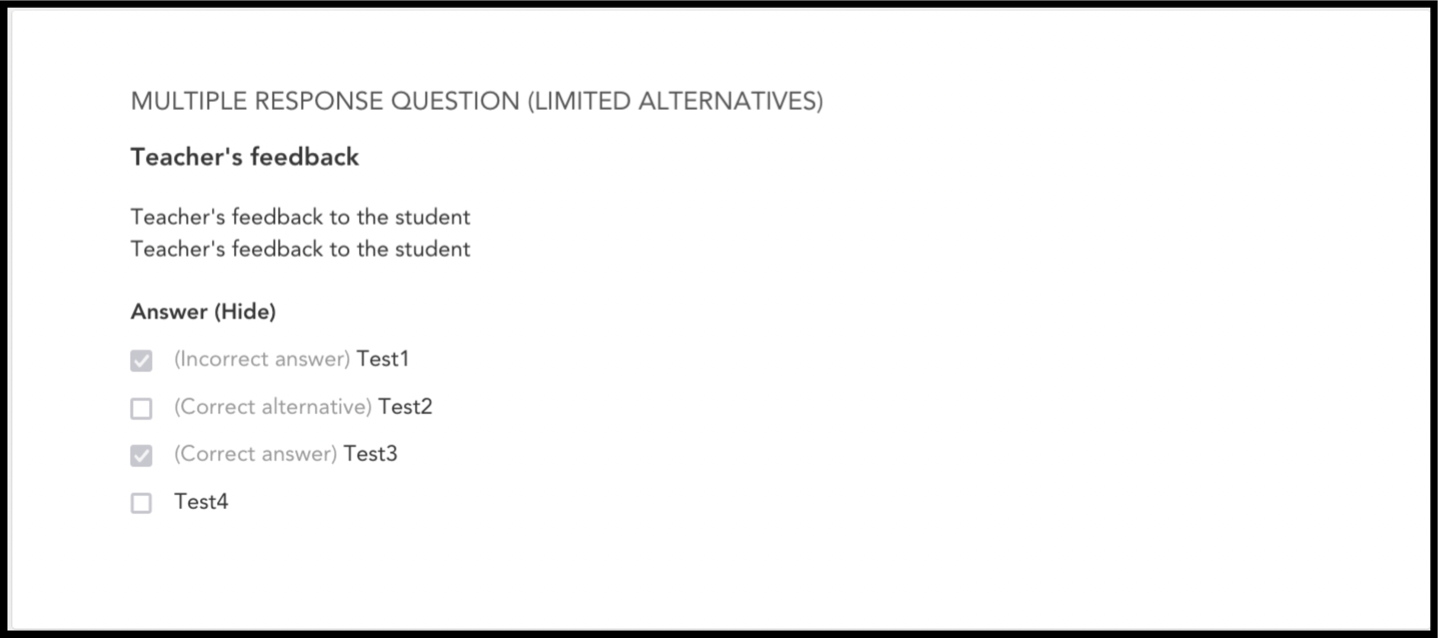Figure 13Figure 14

Example three:

In this example, students can only see the questions on the test and the correct answer. They can not see what they themselves answered.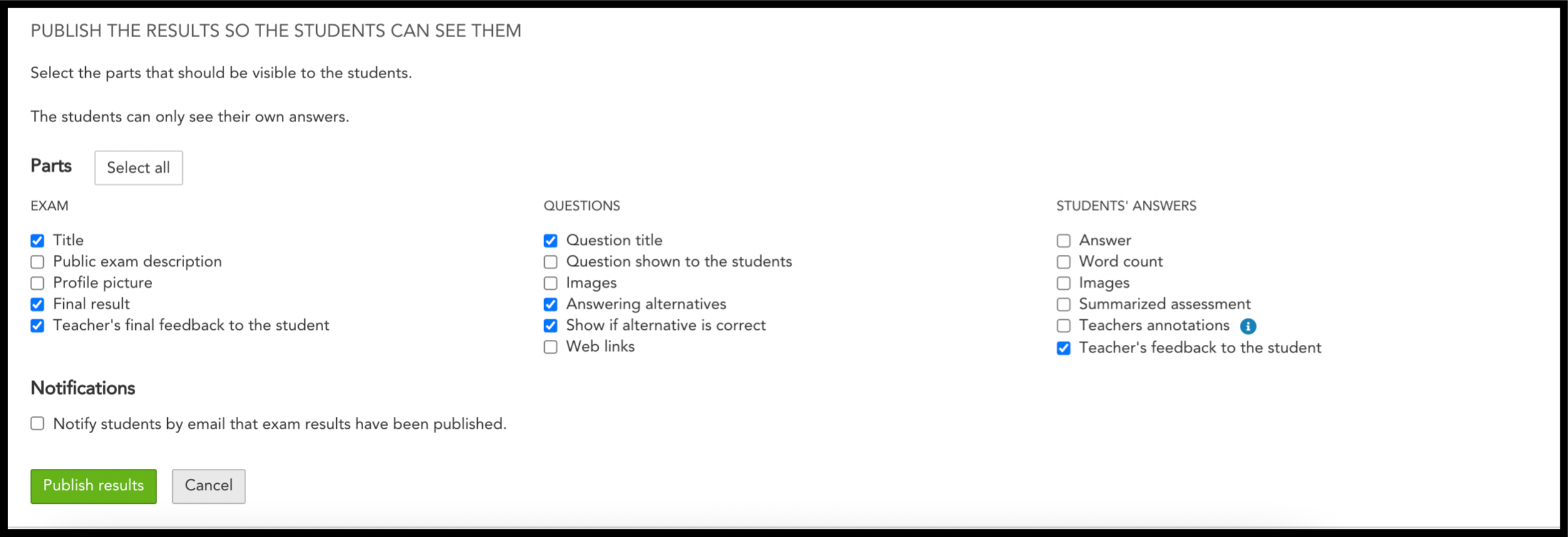Figure 15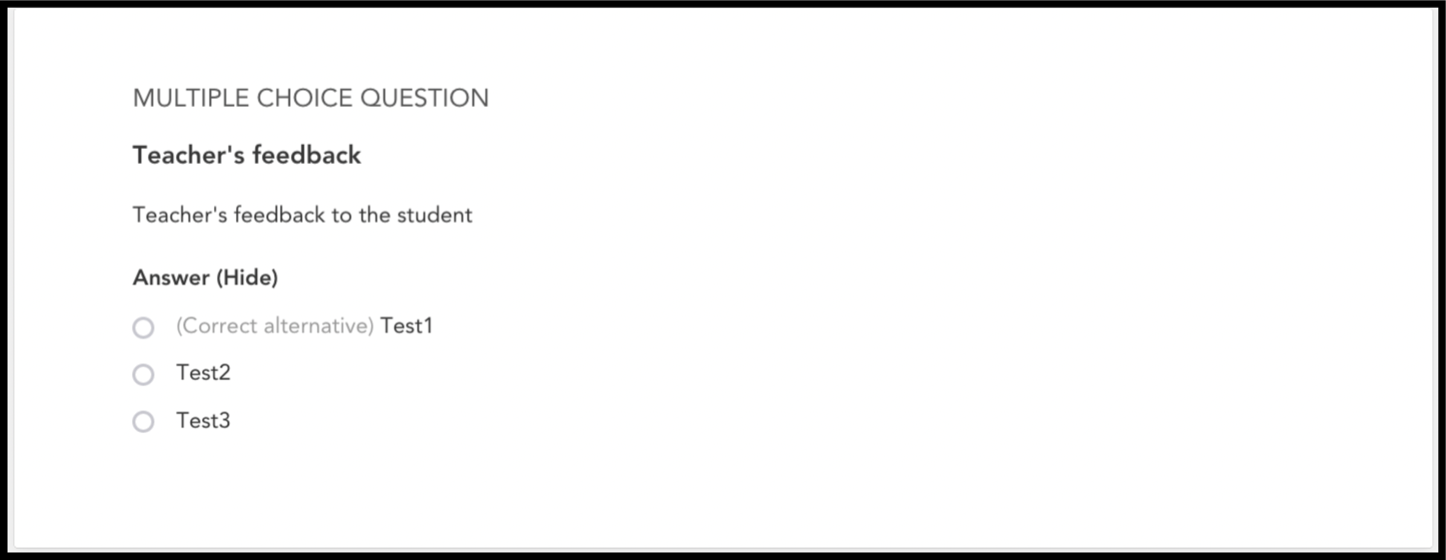Figure 16